# How to Start and Run MATLAB Simulink model? [with Example]

MATLAB Simulink is easier to learn basic building block design for an electrical circuit. They are useful for creating a new model of the system. Various parts of the system are connected to each other to form a simple model.

Simulink has very useful libraries which have different components with a different function to build the model. MATLAB has various versions based on different functions.As you are reading this article, I know, you are new to the MATLAB software. And you may have many doubts. I will explain everything you need to start with MATLAB simulation.

It will be fun when I will demonstrate you a simple example of creating a Sin wave Simulink model.

Let’s begin…

### How to start and Run MATLAB Simulink Model?

At the end of this post, you will learn to run a Simulink model in your MATLAB software.

Hope you have Matlab installed on your system.

#### 1. After Installation and Setup

On my system, I have installed and set up MATLAB version R2013a.

Open MATLAB software and then click on the Simulink library (which is located at the top).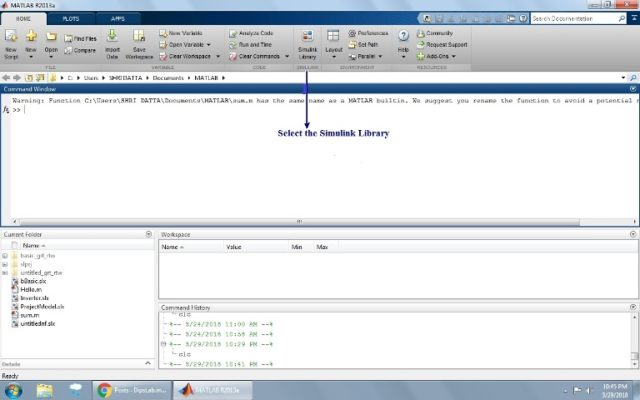####2. Introduction of  Simulink libraries

Simulink library is the collection of the variety of electrical toolbox, source, equipment, load, systems, panel, scope, etc… We need them to build our model.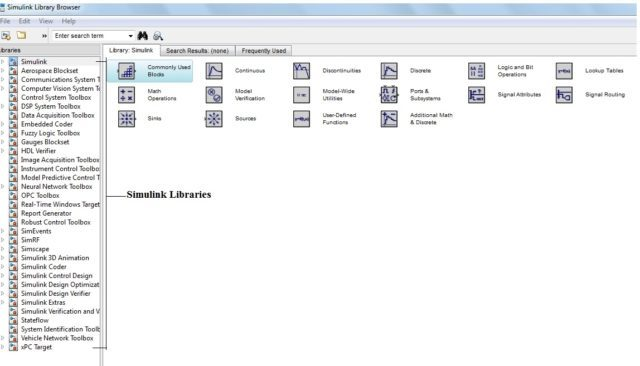#### 3. Creating a New Model

Now actual work begins.

Select new model from the file in Simulink library browser (located in the left sidebar). Here, you need to choose components as per your requirements to build your model.

#### 4. Stateflow of Model

To build a model, you can simply drag and drop the component in the new model. And connect them.

Click on the Run button. You will be notified if there are any errors in your model. Otherwise, everything is fine with your model.

For Example, The considering Sinewave Model.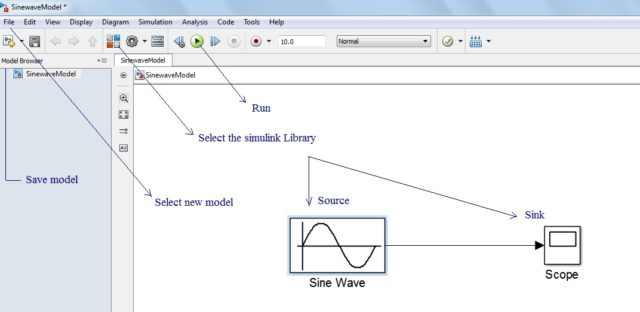#### 5. Save File

By default, this new model saves in the user document folder on your computer. The file extension of the model is a ‘.mdl’.

#### 6. Output/ Result

Lastly, you can see the output and result of your Simulink model by pressing the double click on the scope.

Let’s see the example to demonstrate all our steps.

### Sin Wave Model using MATLAB Simulink

In this example, we will draw a simple sin wave model in MATLAB Simulink. And then we will see to run MATLAB Simulink model for sin wave.

In the following image, you can see, a single sin wave (source) is connected to the scope (sink) by a straight arrow line.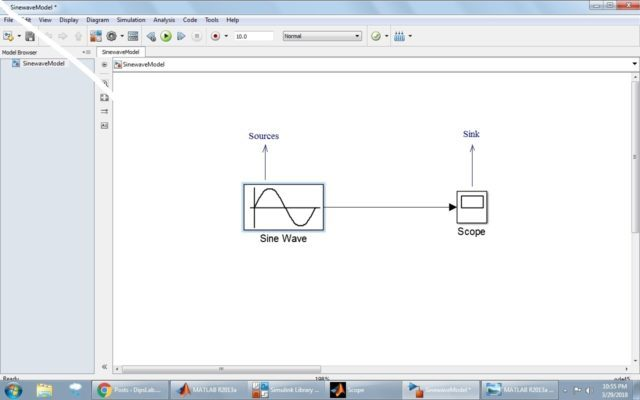Figure 1: Input of Sin wave

When you double-click on the source, you can see the source block parameter. This helps to select several choices related to the terms.

The changing of the parameter also changes its output result.Figure 2: Parameter of source

Scope or sink represents the output result in the form of the wave.

When you select the setting option,  you can set the different function like as General, History, Style for result or output.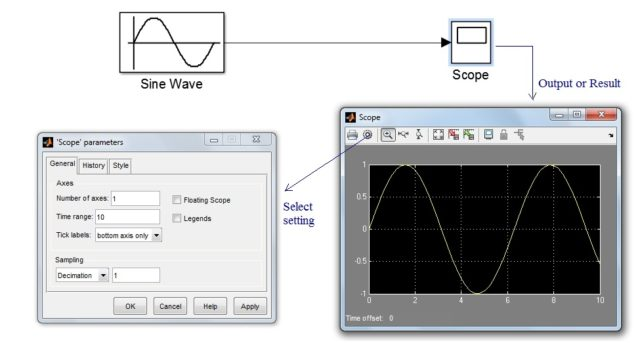Figure 3: Output of Sin wave

Finally, we got the output of sin wave with the peak value of amplitude and periodic time ‘t’.

These are some proper steps to run MATLAB Simulink model. I know you may still some doubt as you are new to the MATLAB. Feel free to ask any doubt in the comment. I will reply you back.

If You Appreciate What I Do Here On DipsLab, You Should Consider:

DipsLab is the fastest growing and most trusted community site for Electrical and Electronics Engineers. All the published articles are available FREELY to all.

If you like what you are reading, please consider buying me a coffee ( or 2 ) as a token of appreciation.

This will help me to continue my services and pay the bills.I’m thankful for your never-ending support.

### 2 thoughts on “How to Start and Run MATLAB Simulink model? [with Example]”

1.Very nice information on MATLAB.

•